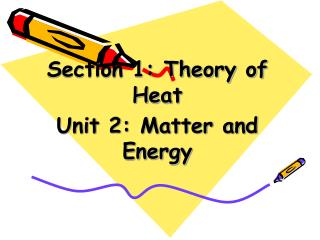# Section 1: Theory of Heat Unit 2: Matter and Energy - PowerPoint PPT PresentationDownload PresentationSection 1: Theory of Heat Unit 2: Matter and Energy

Section 1: Theory of Heat Unit 2: Matter and Energy
Download Presentation## Section 1: Theory of Heat Unit 2: Matter and Energy

- - - - - - - - - - - - - - - - - - - - - - - - - - - E N D - - - - - - - - - - - - - - - - - - - - - - - - - - -
##### Presentation Transcript

1. Section 1: Theory of Heat Unit 2: Matter and Energy

2. Unit Objectives After studying this chapter, you should be able to: • Define matter and identify its states • Identify and describe common gas laws • Define specific gravity and specific volume • Describe the concepts of work and power • Define horsepower • Convert horsepower to watts and btus

3. MATTER • Described as any substance that occupies space and has mass • Matter is made up of atoms • Exists as a solid, liquid or a gas • The state of matter is determined by heat content of the matter as well as the amount of pressure exerted on the substance

4. All of the force exerted by solids is in the downward direction Solids Molecules in solids have a great attraction for each other and can maintain a definite shape

5. Liquids will take the form and shape of the container in which it is placed Liquids The strength of molecular attraction is lower in liquids than in solids The force of a liquid is exerted outward… … and downward

6. Gases Gases will take the shape of the vessel they are contained in and will completely fill the vessel The pressure of a gas is exerted outward in all directions In the case of a toy balloon… …as it blown up, the pressure causes the balloon to expand or inflate

7. IMPORTANT DEFINITIONS • Mass – Property of matter that responds to gravity • Weight – Depends on the strength of gravitational attraction (More gravity = More weight) • Density – Mass to volume relationship (Water has a density of 62.4 lb/ft3 • Specific Gravity – Density of a substance divided by the density of water • Specific Volume – Volume of one pound of a gas (Measured in ft3/lb)

8. SPECIFIC GRAVITY EXAMPLE • Density of water = 62.4 lb/ft3 • Density of Aluminum = 171 lb/ft3 • Specific gravity of aluminum = Density of aluminum / Density of water = (171 lb/ft3)/(62.4 lb/ft3) = 2.74 • Specific gravity is unitless

9. GAS LAWS • General Law of Perfect Gases – Relates pressure, volume and temperature • Boyle’s Law – Relates pressure and volume • Charles’ Law – Relates volume and temperature • Dalton’s Law – Relates pressures of gases in a mixture • Always use absolute pressures and temperatures when working with gas laws

10. BOYLE’S LAW P1 x V1 = P2 x V2 Where P1 = Initial Pressure P2 = Final Pressure T1 = Initial Temperature T2 = Final Temperature V1 = Initial Volume V2 = Final Volume

11. Boyle’s Law Volume = 24 in3 Pressure = 50 psia Volume = 30 in3 Pressure = 40 psia As the volume decreases, the gas pressure increases

12. CHARLES’ LAW T1 T2 V1 = V2 If V1 = 2000 ft3, T1 = 535R and T2 = 590R, we get: V2 = (V1 x T2)/ T1 = (2000 ft3 x 590 R)/ 535 R V2 = 1,180,000 / 535 = 2205.6 ft3

13. Dalton’s Law P = 30 psig P = 70 psig P = 40 psig Total pressure of a gaseous mixture is the sum of the individual pressures

14. ENERGY • Electrical energy drives motors and pumps in air conditioning systems • Heat energy provides comfort heating and flows from a warmer substance to a cooler substance • Energy cannot be created or destroyed, but can be converted from one type to another • Electrical energy purchased by the kWh, fuel oil by the gallon, natural gas by the cubic foot

15. WORK • Work = Force x Distance • Force is given in pounds, distance in feet • The units of work are foot-pounds, ft-lbs Example: How much work is done to move a 150-pound object 100 feet? Work = Force x Distance = 150 pounds x 100 feet Work = 150 x 100 = 15,000 ft-lbs

16. POWER • The rate at which work is done • Work per unit time, ft-lbs/min • Rated in horsepower • 1 hp = 33,000 ft-lbs/min • Electrical power measured in watts • 1 hp = 746 watts • 1 watt = 3.413 btu • 1 kw = 3,413 btu

17. UNIT SUMMARY • Matter can be in the form of solids, liquids and gases • Specific gravity compares the density of substances • Gas laws relate pressure, volume and temperature • Electrical and heat energy are common in the industry • Work is defined as FORCE times DISTANCE • Power = Work per unit time (Horsepower) • 1 Horsepower = 746 watts • 1 Watt = 3.413 Btu